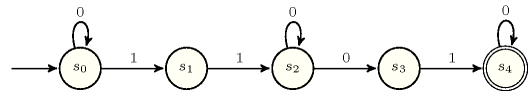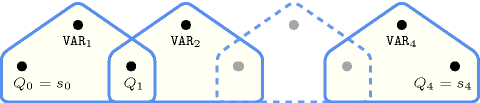## 5.87. consecutive_groups_of_ones

Origin
Constraint

$\mathrm{𝚌𝚘𝚗𝚜𝚎𝚌𝚞𝚝𝚒𝚟𝚎}_\mathrm{𝚐𝚛𝚘𝚞𝚙𝚜}_\mathrm{𝚘𝚏}_\mathrm{𝚘𝚗𝚎𝚜}\left(\mathrm{𝙶𝚁𝙾𝚄𝙿}_\mathrm{𝚂𝙸𝚉𝙴𝚂},\mathrm{𝚅𝙰𝚁𝙸𝙰𝙱𝙻𝙴𝚂}\right)$

Arguments
 $\mathrm{𝙶𝚁𝙾𝚄𝙿}_\mathrm{𝚂𝙸𝚉𝙴𝚂}$ $\mathrm{𝚌𝚘𝚕𝚕𝚎𝚌𝚝𝚒𝚘𝚗}\left(\mathrm{𝚗𝚋}-\mathrm{𝚒𝚗𝚝}\right)$ $\mathrm{𝚅𝙰𝚁𝙸𝙰𝙱𝙻𝙴𝚂}$ $\mathrm{𝚌𝚘𝚕𝚕𝚎𝚌𝚝𝚒𝚘𝚗}\left(\mathrm{𝚟𝚊𝚛}-\mathrm{𝚍𝚟𝚊𝚛}\right)$
Restrictions
 $\mathrm{𝚛𝚎𝚚𝚞𝚒𝚛𝚎𝚍}$$\left(\mathrm{𝙶𝚁𝙾𝚄𝙿}_\mathrm{𝚂𝙸𝚉𝙴𝚂},\mathrm{𝚗𝚋}\right)$ $|\mathrm{𝙶𝚁𝙾𝚄𝙿}_\mathrm{𝚂𝙸𝚉𝙴𝚂}|\ge 1$ $\mathrm{𝙶𝚁𝙾𝚄𝙿}_\mathrm{𝚂𝙸𝚉𝙴𝚂}.\mathrm{𝚗𝚋}\ge 1$ $\mathrm{𝙶𝚁𝙾𝚄𝙿}_\mathrm{𝚂𝙸𝚉𝙴𝚂}.\mathrm{𝚗𝚋}\le |\mathrm{𝚅𝙰𝚁𝙸𝙰𝙱𝙻𝙴𝚂}|$ $\mathrm{𝚛𝚎𝚚𝚞𝚒𝚛𝚎𝚍}$$\left(\mathrm{𝚅𝙰𝚁𝙸𝙰𝙱𝙻𝙴𝚂},\mathrm{𝚟𝚊𝚛}\right)$ $|\mathrm{𝚅𝙰𝚁𝙸𝙰𝙱𝙻𝙴𝚂}|\ge 2*|\mathrm{𝙶𝚁𝙾𝚄𝙿}_\mathrm{𝚂𝙸𝚉𝙴𝚂}|-1$ $|\mathrm{𝚅𝙰𝚁𝙸𝙰𝙱𝙻𝙴𝚂}|\ge$$\mathrm{𝚜𝚞𝚖}$$\left(\mathrm{𝙶𝚁𝙾𝚄𝙿}_\mathrm{𝚂𝙸𝚉𝙴𝚂}.\mathrm{𝚗𝚋}\right)+|\mathrm{𝙶𝚁𝙾𝚄𝙿}_\mathrm{𝚂𝙸𝚉𝙴𝚂}|-1$ $\mathrm{𝚅𝙰𝚁𝙸𝙰𝙱𝙻𝙴𝚂}.\mathrm{𝚟𝚊𝚛}\ge 0$ $\mathrm{𝚅𝙰𝚁𝙸𝙰𝙱𝙻𝙴𝚂}.\mathrm{𝚟𝚊𝚛}\le 1$
Purpose

In order to define the meaning of the $\mathrm{𝚌𝚘𝚗𝚜𝚎𝚌𝚞𝚝𝚒𝚟𝚎}_\mathrm{𝚐𝚛𝚘𝚞𝚙𝚜}_\mathrm{𝚘𝚏}_\mathrm{𝚘𝚗𝚎𝚜}$ constraint, we first introduce the notions of stretch and span. Let $n$ be the number of variables of the collection $\mathrm{𝚅𝙰𝚁𝙸𝙰𝙱𝙻𝙴𝚂}$ and let $m$ be the number of items of the collection $\mathrm{𝙶𝚁𝙾𝚄𝙿}_\mathrm{𝚂𝙸𝚉𝙴𝚂}$. Let ${X}_{i},\cdots ,{X}_{j}$ $\left(1\le i\le j\le n\right)$ be consecutive variables of the collection of variables $\mathrm{𝚅𝙰𝚁𝙸𝙰𝙱𝙻𝙴𝚂}$ such that the following conditions apply:

• All variables ${X}_{i},\cdots ,{X}_{j}$ are assigned value 1,

• $i=1$ or ${X}_{i-1}\ne 1$,

• $j=n$ or ${X}_{j+1}\ne 1$.

We call such a set of variables a stretch. The span of the stretch is equal to $j-i+1$. We now define the condition enforced by the $\mathrm{𝚌𝚘𝚗𝚜𝚎𝚌𝚞𝚝𝚒𝚟𝚎}_\mathrm{𝚐𝚛𝚘𝚞𝚙𝚜}_\mathrm{𝚘𝚏}_\mathrm{𝚘𝚗𝚎𝚜}$ constraint.

All variables of the $\mathrm{𝚅𝙰𝚁𝙸𝙰𝙱𝙻𝙴𝚂}$ collection should be assigned value 0 or 1. In addition there is $|\mathrm{𝙶𝚁𝙾𝚄𝙿}_\mathrm{𝚂𝙸𝚉𝙴𝚂}|$ successive stretches of respective span $\mathrm{𝙶𝚁𝙾𝚄𝙿}_\mathrm{𝚂𝙸𝚉𝙴𝚂}\left[1\right].\mathrm{𝚗𝚋},$ $\mathrm{𝙶𝚁𝙾𝚄𝙿}_\mathrm{𝚂𝙸𝚉𝙴𝚂}\left[2\right].\mathrm{𝚗𝚋},\cdots ,$ $\mathrm{𝙶𝚁𝙾𝚄𝙿}_\mathrm{𝚂𝙸𝚉𝙴𝚂}\left[m\right].\mathrm{𝚗𝚋}$.

Example
$\left(〈2,1〉,〈1,1,0,0,0,1,0〉\right)$

The $\mathrm{𝚌𝚘𝚗𝚜𝚎𝚌𝚞𝚝𝚒𝚟𝚎}_\mathrm{𝚐𝚛𝚘𝚞𝚙𝚜}_\mathrm{𝚘𝚏}_\mathrm{𝚘𝚗𝚎𝚜}$ constraint holds since the sequence $1100010$ contains a first stretch (i.e., a maximum sequence of 1) of span 2 and a second stretch of span 1.

Typical
 $|\mathrm{𝚅𝙰𝚁𝙸𝙰𝙱𝙻𝙴𝚂}|>1$ $\mathrm{𝚛𝚊𝚗𝚐𝚎}$$\left(\mathrm{𝚅𝙰𝚁𝙸𝙰𝙱𝙻𝙴𝚂}.\mathrm{𝚟𝚊𝚛}\right)>1$
Symmetry

Items of $\mathrm{𝙶𝚁𝙾𝚄𝙿}_\mathrm{𝚂𝙸𝚉𝙴𝚂}$ and $\mathrm{𝚅𝙰𝚁𝙸𝙰𝙱𝙻𝙴𝚂}$ are simultaneously reversable.

Usage

The $\mathrm{𝚌𝚘𝚗𝚜𝚎𝚌𝚞𝚝𝚒𝚟𝚎}_\mathrm{𝚐𝚛𝚘𝚞𝚙𝚜}_\mathrm{𝚘𝚏}_\mathrm{𝚘𝚗𝚎𝚜}$ constraint can be used in order to model the logigraphe problem.

Figure 5.87.1 depicts the automaton associated with the $\mathrm{𝚌𝚘𝚗𝚜𝚎𝚌𝚞𝚝𝚒𝚟𝚎}_\mathrm{𝚐𝚛𝚘𝚞𝚙𝚜}_\mathrm{𝚘𝚏}_\mathrm{𝚘𝚗𝚎𝚜}$ constraint. To each variable ${\mathrm{𝚅𝙰𝚁}}_{i}$ of the collection $\mathrm{𝚅𝙰𝚁𝙸𝙰𝙱𝙻𝙴𝚂}$ corresponds a signature variable that is equal to ${\mathrm{𝚅𝙰𝚁}}_{i}$. There is no signature constraint.
##### Figure 5.87.1. Non deterministic automaton of the $\mathrm{𝚌𝚘𝚗𝚜𝚎𝚌𝚞𝚝𝚒𝚟𝚎}_\mathrm{𝚐𝚛𝚘𝚞𝚙𝚜}_\mathrm{𝚘𝚏}_\mathrm{𝚘𝚗𝚎𝚜}$ constraint of the Example slot (a stretch of two 1 followed by a stretch of a single 1)##### Figure 5.87.2. Hypergraph of the reformulation corresponding to the automaton of the $\mathrm{𝚌𝚘𝚗𝚜𝚎𝚌𝚞𝚝𝚒𝚟𝚎}_\mathrm{𝚐𝚛𝚘𝚞𝚙𝚜}_\mathrm{𝚘𝚏}_\mathrm{𝚘𝚗𝚎𝚜}$ constraint of the Example slot